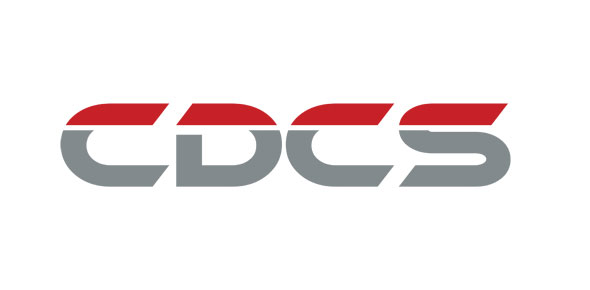# 2A551 CDCs Volume 3 Of 3 Ure Questions Only

100 Questions | Attempts: 1079Settings• 1.
It is important to raise the air temperature in a jet engine to
• A.

Increase its energy

• B.

Decrease its energy

• C.

Decrease its volume

• D.

Increase its efficiency

• 2.
In a jet engine, the velocity of the air is decreased by the
• A.

Diffuser

• B.

Afterburner

• C.

Compressor

• D.

• 3.
An aircraft taxiing at a constant speed is an example of
• A.

Bernoulli's Law

• B.

Newton's First Law of Motion

• C.

Newton's Second Law of Motion

• D.

Newton's Third Law of Motion

• 4.
Foot-pounds and inch-pounds are units of measure for
• A.

Work

• B.

Power

• C.

Inertia

• D.

Friction

• 5.
The resistance of an object to change its motion is a property of matter called
• A.

Energy

• B.

Inertia

• C.

Friction

• D.

Potential

• 6.
The ability to do work is the definition of
• A.

Energy

• B.

Inertia

• C.

Friction

• D.

Velocity

• 7.
In a jet engine, fuel is metered for combustion by the
• A.

Pressurizing and dump (P&D) valve

• B.

Fuel nozzles

• C.

Fuel control

• D.

Fuel pump

• 8.
The average percentage of a jet engine's energy required to maintain engine operation is
• A.

50

• B.

60

• C.

70

• D.

80

• 9.
What effect does the divergent design of a jet engine diffuser have on pressure?
• A.

Increases pressure

• B.

Decreases pressure

• C.

Results in pressure fluctuations

• D.

Has minimum effect on pressure

• 10.
The required velocity for air and gases flowing through a jet engine is
• A.

Low velocity at all times

• B.

Less velocity exiting than entering

• C.

Equal velocity entering and exiting

• D.

Greater velocity exiting than entering

• 11.
The velocity of the stream of air that flows through a jet engine reaches its highest point at the
• A.

Turbine stator (nozzle diaphragm)

• B.

Turbine exhaust cone

• C.

Combustion section

• D.

Ejector nozzle

• 12.
When two or more turbine wheels are used in a jet engine, what is placed directly in front of each turbine wheel?
• A.

Diffuser

• B.

Jet nozzle

• C.

Combustion liner

• D.

Nozzle diaphragm

• 13.
A jet engine derives its name from the fact that
• A.

It is in effect a turbo-supercharger

• B.

The nozzles within the engine are called jets

• C.

It uses a turbine-type compressor to maintain power

• D.

It uses a exhaust-gas-driven turbine wheel to drive its compressor

• 14.
The three major sections of all jet engines are compressor, combustion, and
• A.

Turbo

• B.

Turbine

• C.

Exhaust

• D.

Diffuser

• 15.
The condition that rapidly reduces the efficiency of a centrifugal jet engine compressor would be
• A.

Resultant velocity

• B.

Tangential velocity

• C.

Pressure pulsations

• D.

Air mass leaving the impeller at great velocities

• 16.
The type of engine that is cheaper to manufacture is the
• A.

Axial-flow because of size

• B.

Centrifugal because of size

• C.

Axial-flow because of fewer parts

• D.

Centrifugal because of fewer parts

• 17.
The percentage of combustion efficiency of a gas turbine is usually between
• A.

60 and 70

• B.

65 and 75

• C.

75 and 95

• D.

95 and 100

• 18.
The term "combustion range" is defined as a jet engine's
• A.

• B.

Pressure-ratio zone

• C.

Compressor stall zone

• D.

Operating temperature zone

• 19.
The most chemically correct ratio for burning fuel in a combustion chamber is
• A.

10:1

• B.

10:4

• C.

15:1

• D.

15:4

• 20.
The inner and outer surfaces of an annular-type combustion chamber are formed by
• A.

Interlocking stainless steel bands

• B.

The inner and outer diffuser case

• C.

A forged steel casing

• D.

Solid sheet metal

• 21.
The most common type of fuel nozzle system is the
• A.

Pressure-atomizing

• B.

Fuel-blasting

• C.

Fuel ejector

• D.

Fuel tube

• 22.
Swirl-type fuel nozzles are usually used to provide what type of flame speed?
• A.

Low

• B.

High

• C.

Medium

• D.

Medium-high

• 23.
The three design types of turbines used in jet engines are
• A.

Shrouded, unshrouded, and fir tree

• B.

Shrouded, unshrouded, and corrugated

• C.

Impulse, reaction, and reaction-impulse

• D.

Impeller, impulse, reaction-impulse

• 24.
On some jet engines, the turbine bucket is secured in place by
• A.

Screws

• B.

Cables

• C.

Lockwire

• D.

Lock strips

• 25.
The purpose of the exhaust duct is to
• A.

Swirl exhaust gas-flow

• B.

Equalize exhaust gas-flow

• C.

Modulate exhaust gas-flow

• D.

Straighten exhaust gas-flow

## Related TopicsBack to top
×

Wait!
Here's an interesting quiz for you.